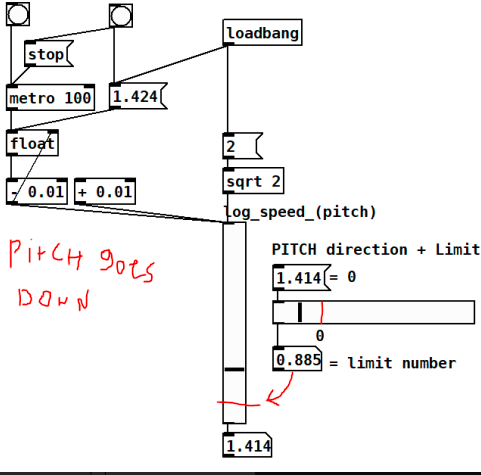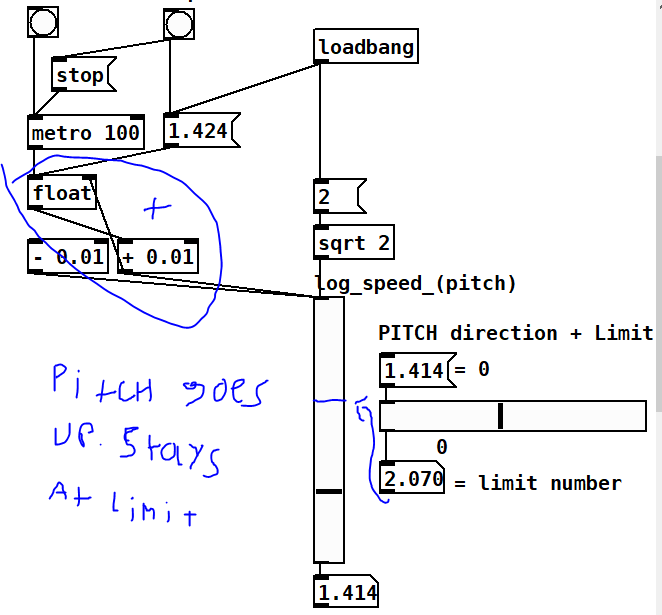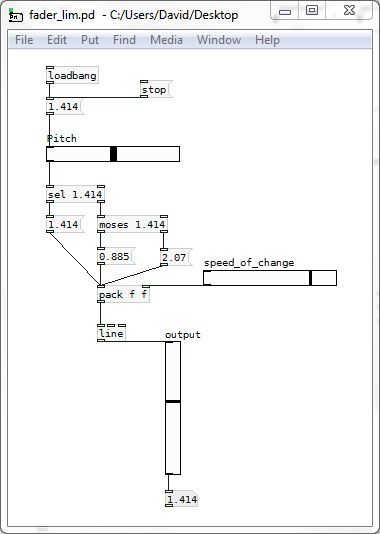• How to make a slider have a limit and change direction?

Ok, here's a better explanation. 4.414 is zero (value at which the pitch of my sample is unaffected). I want to have a slider that can go either above 4.414 or below to set a limit. If the number is under 4.414 the number decreases going below up to that limit number (in this example limit is 0.885)

And I'd like it to do the opposite direction, so that if the slider is above 4.414 the number would increase up to the limit, (on this second example 2.070)

Once it hits the limit stays at that value until. I press stop and it resets.

• | Posts 3 | Views 78
•